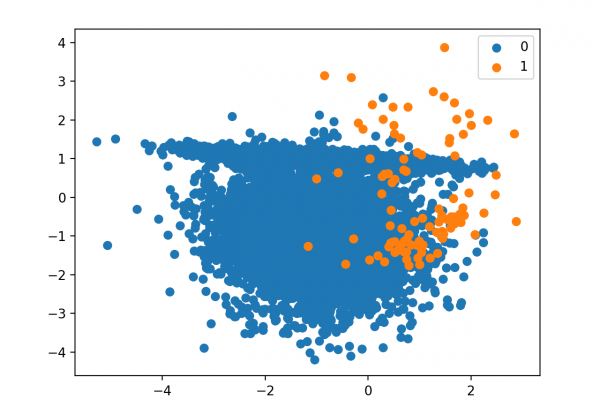# How to Develop a Cost-Sensitive Neural Network for Imbalanced ClassificationLast Updated on August 21, 2020

Deep learning neural networks are a flexible class of machine learning algorithms that perform well on a wide range of problems.

Neural networks are trained using the backpropagation of error algorithm that involves calculating errors made by the model on the training dataset and updating the model weights in proportion to those errors. The limitation of this method of training is that examples from each class are treated the same, which for imbalanced datasets means that the model is adapted a lot more for one class than another.

The backpropagation algorithm can be updated to weigh misclassification errors in proportion to the importance of the class, referred to as weighted neural networks or cost-sensitive neural networks. This has the effect of allowing the model to pay more attention to examples from the minority class than the majority class in datasets with a severely skewed class distribution.

In this tutorial, you will discover weighted neural networks for imbalanced classification.

After completing this tutorial, you will know:

• How the standard neural network algorithm does not support imbalanced classification.
• How the neural network training algorithm can be modified to weight misclassification errors in proportion to